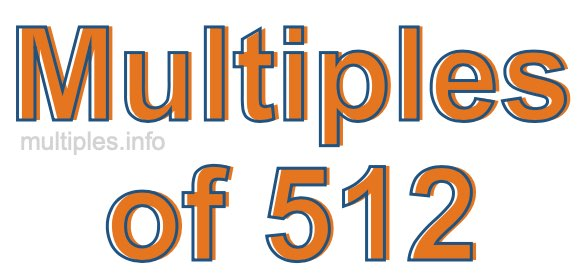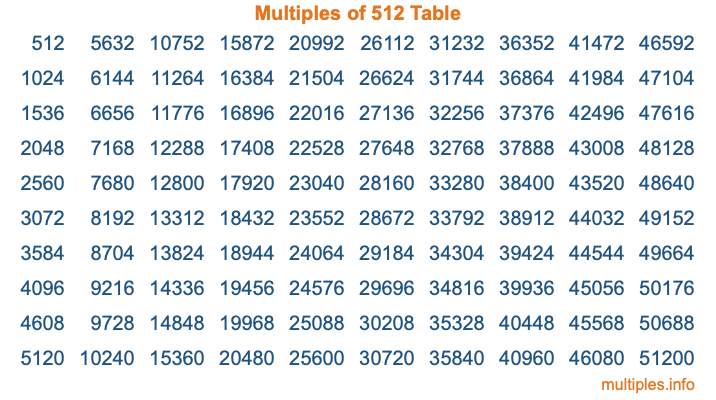Multiples of 512Welcome to the Multiples of 512 page. Here we will first teach you everything you will ever need to know about the multiples of 512, and then give you a study guide summary of everything we taught you to make sure you remember it all. Use this page to look up facts and learn information about the multiples of 512. This page will make you a multiples of five hundred twelve expert!

Definition of Multiples of 512
Multiples of 512 are all the numbers that when divided by 512 equal an integer. Each of the multiples of 512 are called a multiple. A multiple of 512 is created by multiplying 512 by an integer.

Therefore, to create a list of multiples of 512, you start with 1 multiplied by 512, then 2 multiplied by 512, then 3 multiplied by 512, and so on for as long as you want. Thus, the list of the first five multiples of 512 is 512, 1024, 1536, 2048, and 2560. To see a larger list of multiples of 512, see the printable image of Multiples of 512 further down on this page. We also have a category where you can choose any nth multiple of 512.

Multiples of 512 Checker
The Multiples of 512 Checker below checks to see if any number of your choice is a multiple of 512. In other words, it checks to see if there is any number (integer) that when multiplied by 512 will equal your number. To do that, we divide your number by 512. If the the quotient is an integer, then your number is a multiple of 512.

Is  a multiple of 512?

Least Common Multiple of 512 and ...
A Least Common Multiple (LCM) is the lowest multiple that two or more numbers have in common. This is also called the smallest common multiple or lowest common multiple and is useful to know when you are adding our subtracting fractions. Enter one or more numbers below (512 is already entered) to find the LCM.

Check out our LCM Calculator if you need more details about the Least Common Multiple or if you need the LCM for different numbers for adding and subtraction fractions.

nth Multiple of 512
As we stated above, 512 is the first multiple of 512, 1024 is the second multiple of 512, 1536 is the third multiple of 512, and so on. Enter a number below to find the nth multiple of 512.

th multiple of 512

Multiples of 512 vs Factors of 512
512 is a multiple of 512 and a factor of 512, but that is where the similarities end. All postive multiples of 512 are 512 or greater than 512. All positive factors of 512 are 512 or less than 512.

Below is the beginning list of multiples of 512 and the factors of 512 so you can compare:

Multiples of 512: 512, 1024, 1536, 2048, 2560, etc.

Factors of 512: 1, 2, 4, 8, 16, 32, 64, 128, 256, 512

As you can see, the multiples of 512 are all the numbers that you can divide by 512 to get a whole number. The factors of 512, on the other hand, are all the whole numbers that you can multiply by another whole number to get 512.

It's also interesting to note that if a number (x) is a factor of 512, then 512 will also be a multiple of that number (x).

Multiples of 512 vs Divisors of 512
The divisors of 512 are all the integers that 512 can be divided by evenly. Below is a list of the divisors of 512.

Divisors of 512: 1, 2, 4, 8, 16, 32, 64, 128, 256, 512

The interesting thing to note here is that if you take any multiple of 512 and divide it by a divisor of 512, you will see that the quotient is an integer.

Multiples of 512 Table
Below is an image of the first 100 multiples of 512 in a table. The table is in chronological order, column by column. The first column has the first ten multiples of 512, the second column has the next ten multiples of 512, and so on.The Multiples of 512 Table is also referred to as the 512 Times Table or Times Table of 512. You are welcome to print out our table for your studies.

Negative Multiples of 512
Although not often discussed or needed in math, it is worth mentioning that you can make a list of negative multiples of 512 by multiplying 512 by -1, then by -2, then by -3, and so on, to get the following list of negative multiples of 512:

-512, -1024, -1536, -2048, -2560, etc.

Multiples of 512 Summary
Below is a summary of important Multiples of 512 facts that we have discussed on this page. To retain the knowledge on this page, we recommend that you read through the summary and explain to yourself or a study partner why they hold true.

There are an infinite number of multiples of 512.

A multiple of 512 divided by 512 will equal a whole number.

512 divided by a factor of 512 equals a divisor of 512.

The nth multiple of 512 is n times 512.

The largest factor of 512 is equal to the first positive multiple of 512.

512 is a multiple of every factor of 512.

512 is a multiple of 512.

A multiple of 512 divided by a divisor of 512 equals an integer.

512 divided by a divisor of 512 equals a factor of 512.

Any integer times 512 will equal a multiple of 512.

Multiples of a Number
Here you can get the multiples of another number, all with the same attention to detail as we did for multiples of 512 on this page.

Multiples of
Multiples of 513
Did you find our page about multiples of five hundred twelve educational? Do you want more knowledge? Check out the multiples of the next number on our list!